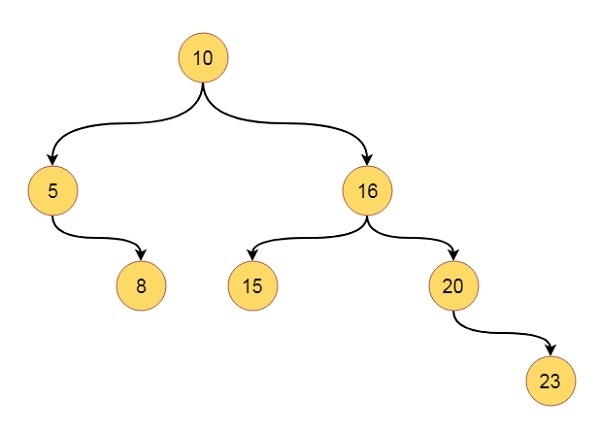# Binary Search Trees in Data Structures

The binary search trees are binary tree which has some properties. These properties are like below −

• Every Binary Search Tree is a binary tree
• Every left child will hold lesser value than root
• Every right child will hold greater value than root
• Ideal binary search tree will not hold same value twice.

Suppose we have one tree like this −This tree is one binary search tree. It follows all of the mentioned properties. If we traverse elements into inorder traversal mode, we can get 5, 8, 10, 15, 16, 20, 23. Let us see one code to understand how we can implement this in C++ code.

## Example

Live Demo

#include<iostream>
using namespace std;
class node{
public:
int h_left, h_right, bf, value;
node *left, *right;
};
class tree{
private:
node *get_node(int key);
public:
node *root;
tree(){
root = NULL; //set root as NULL at the beginning
}
void inorder_traversal(node *r);
node *insert_node(node *root, int key);
};
node *tree::get_node(int key){
node *new_node;
new_node = new node; //create a new node dynamically
new_node->h_left = 0; new_node->h_right = 0;
new_node->bf = 0;
new_node->value = key; //store the value from given key
new_node->left = NULL; new_node->right = NULL;
return new_node;
}
void tree::inorder_traversal(node *r){
if(r != NULL){ //When root is present, visit left - root - right
inorder_traversal(r->left);
cout << r->value << " ";
inorder_traversal(r->right);
}
}
node *tree::insert_node(node *root, int key){
if(root == NULL){
return (get_node(key)); //when tree is empty, create a node as root
}
if(key < root->value){ //when key is smaller than root value, go to the left
root->left = insert_node(root->left, key);
}else if(key > root->value){ //when key is greater than root value, go to the right
root->right = insert_node(root->right, key);
}
return root; //when key is already present, do not insert it again
}
main(){
node *root;
tree my_tree;
//Insert some keys into the tree.
my_tree.root = my_tree.insert_node(my_tree.root, 10);
my_tree.root = my_tree.insert_node(my_tree.root, 5);
my_tree.root = my_tree.insert_node(my_tree.root, 16);
my_tree.root = my_tree.insert_node(my_tree.root, 20);
my_tree.root = my_tree.insert_node(my_tree.root, 15);
my_tree.root = my_tree.insert_node(my_tree.root, 8);
my_tree.root = my_tree.insert_node(my_tree.root, 23);
cout << "In-Order Traversal: ";
my_tree.inorder_traversal(my_tree.root);
}

## Output

In-Order Traversal: 5 8 10 15 16 20 23